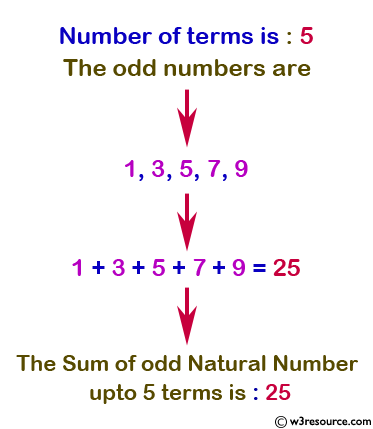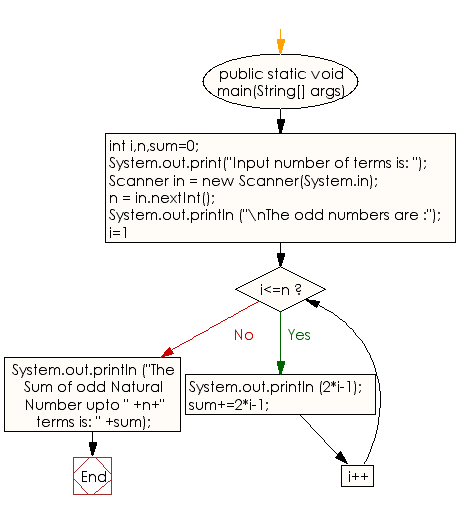﻿ Java exercises: Display the n terms of odd natural number and their sum - w3resource# Java Conditional Statement Exercises: Display the n terms of odd natural number and their sum

## Java Conditional Statement: Exercise-15 with Solution

Write a program in Java to display the n terms of odd natural number and their sum.

Test Data
Input number of terms is: 5

Pictorial Presentation:Sample Solution:

Java Code:

``````import java.util.Scanner;
public class Exercise15 {

public static void main(String[] args)

{
int i,n,sum=0;

System.out.print("Input number of terms is: ");
{
Scanner in = new Scanner(System.in);
n = in.nextInt();
System.out.println ("\nThe odd numbers are :");
for(i=1;i<=n;i++)

{
System.out.println (2*i-1);
sum+=2*i-1;
}
System.out.println ("The Sum of odd Natural Number upto " +n+" terms is: " +sum);

}
}
}
```
```

Sample Output:

```Input number of terms is: 5
The odd numbers are :
1
3
5
7
9
The Sum of odd Natural Number upto 5 terms is: 25
```

Flowchart:Java Code Editor: